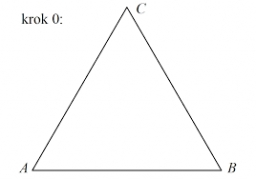# Equilateral 37341

Calculate an equilateral triangle's perimeter and area with a side of 20 dm.

o =  60 dm
S =  173.2051 dm2

### Step-by-step explanation:Did you find an error or inaccuracy? Feel free to write us. Thank you!

Tips for related online calculators
The Pythagorean theorem is the base for the right triangle calculator.
Calculation of an equilateral triangle.